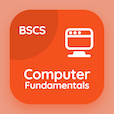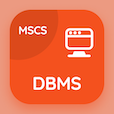Online CS Degree Courses

Computer Fundamentals MCQs

Computer Fundamentals MCQ - Topics

Learn Truth Tables Multiple Choice Questions (MCQ Quiz), Truth Tables quiz answers PDF to learn computer fundamentals course for computer fundamentals online classes. Digital Logic Multiple Choice Questions and Answers (MCQs), Truth Tables quiz questions for computer software engineer online degree. Truth Tables Book PDF: truth tables, logic circuits and logic gates test prep for computer science associate degree.

"Operation carried out by a NOT gate are also termed as" MCQ PDF: truth tables App APK with inverting, converting, reverting, and reversing choices for computer software engineer online degree. Study truth tables quiz questions for merit scholarship test and certificate programs for 2 year computer science degree.

## MCQs on Truth Tables Quiz

MCQ: Operation carried out by a NOT gate are also termed as

inverting
converting
reverting
reversing

MCQ: Logic gate in which output is zero for the inputs in which one input is one and other inputs are zero is classified as

AND gate
NOT gate
OR gate
OUT gate

MCQ: Logic gate in which any one of inputs is logic 1 results in output as logic 1 is termed as

NOT gate
NOR gate
AND gate
OR gate

MCQ: Table used to show the possible combination of inputs for an output is said to be

logic table
gate table
system circuit table
truth table

MCQ: Table that shows the result of logical operations conducted is called

truth table
system table
logic table
circuit table

### More Quizzes on Computer Fundamentals Book